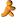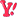Wrox Programmer ForumsVB Macro Excel
 |
 Excel VBA Discuss using VBA for Excel programming.
 Welcome to the p2p.wrox.com Forums. You are currently viewing the Excel VBA section of the Wrox Programmer to Programmer discussions. This is a community of software programmers and website developers including Wrox book authors and readers. New member registration was closed in 2019. New posts were shut off and the site was archived into this static format as of October 1, 2020. If you require technical support for a Wrox book please contact http://hub.wiley.com

 mateenmohdFriend of Wrox Join Date: Jun 2003 Posts: 518 Thanks: 0 Thanked 0 Times in 0 PostsVB Macro Excel

I have two sheets, sheet1 and sheet2
sheet1 is
------------
A B C D
----------------
-----------------
101 121 131 25
102 122 132 362
103 123 133 39
104 124 134 14
105 125 135 37
106 126 136 35
107 127 137 5
108 128 138 39
109 129 139 325
110 130 140 27

sheet2 is
------------
A B C D
---------------
----------------
101 121 131
102 122 132
103 123 133
104 124 134
105 125 135
106 126 136
107 127 137
108 128 138
109 129 139
110 130 140

I have to compare sheet1 fields AD, AF and TO
with sheet2 if AD=AF=TO then write sheet1 DATA value into sheet2
DATA field, how can write the vb marco in excel ?
ie.
I want that check value of sheet1 AF,AD and TO
ie 101=121=131 then write sheet1 DATA field value 25
into sheet2 DATA field how ?
what macro will use ?

Mateen

 vemajuFriend of Wrox Join Date: Oct 2003 Posts: 168 Thanks: 0 Thanked 0 Times in 0 PostsHi Mateen,

You can use this code.
Initialize variable i to the row your table begins

Sub WriteData()
Dim i As Integer
i = 1
Worksheets("Sheet1").Activate
Do Until IsEmpty(Cells(i, 30))
If Cells(i, 30) = Sheets("Sheet2").Cells(i, 30) And _
Cells(i, 31) = Sheets("Sheet2").Cells(i, 31) And _
Cells(i, 32) = Sheets("Sheet2").Cells(i, 32) Then
Sheets("Sheet2").Cells(i, 33) = Sheets("Sheet1").Cells(i, 33)
End If
i = i + 1
Loop
End Sub

-vemaju

 mateenmohdFriend of Wrox Join Date: Jun 2003 Posts: 518 Thanks: 0 Thanked 0 Times in 0 PostsHi, vemaju,

I try to run your macro, but it not write data to sheet2 data colum.
nothing. I could not sucess to write the data to sheet2 data
colum. I create new macro and write the code.
I could not understand where I making mistake.

regards

Mateen

 vemajuFriend of Wrox Join Date: Oct 2003 Posts: 168 Thanks: 0 Thanked 0 Times in 0 PostsHi,

Sorry. The colums are wrong. Here's the right code

Sub WriteData()
Dim i As Integer
i = 1
Worksheets("Sheet1").Activate
Do Until IsEmpty(Cells(i, 1))
If Cells(i, 1) = Sheets("Sheet2").Cells(i, 1) And _
Cells(i, 2) = Sheets("Sheet2").Cells(i, 1) And _
Cells(i, 3) = Sheets("Sheet2").Cells(i, 3) Then
Sheets("Sheet2").Cells(i, 4) = Sheets("Sheet1").Cells(i, 4)
End If
i = i + 1
Loop
End Sub

-vemaju

 mateenmohdFriend of Wrox Join Date: Jun 2003 Posts: 518 Thanks: 0 Thanked 0 Times in 0 PostsMacro working fine. I have thousand of records.
How can display the records to another column
which not match. it need to defined another variable ?
I try this,but it not write data which not match.

Sub writedata()
Dim i As Integer
i = 1
Worksheets("Sheet1").Activate
Do Until IsEmpty(Cells(i, 1))
If Cells(i, 1) = Sheets("Sheet2").Cells(i, 1) And _
Cells(i, 2) = Sheets("Sheet2").Cells(i, 2) And _
Cells(i, 3) = Sheets("Sheet2").Cells(i, 3) Then
Sheets("Sheet2").Cells(i, 4) = Sheets("Sheet1").Cells(i, 4)

End If

i = i + 1
Loop

Dim k As Integer
k = 1
Worksheets("Sheet1").Activate
Do Until IsEmpty(Cells(k, 1))
If Cells(k, 1) <> Sheets("Sheet2").Cells(k, 1) And _
Cells(k, 2) <> Sheets("Sheet2").Cells(k, 2) And _
Cells(k, 3) <> Sheets("Sheet2").Cells(k, 3) Then
Sheets("Sheet2").Cells(k, 5) = Sheets("Sheet1").Cells(k, 4)

End If

k = k + 1
Loop
End Sub

regards

Mateen

 shatteredAuthorized User Join Date: Oct 2004 Posts: 60 Thanks: 0 Thanked 0 Times in 0 PostsYou just need to add an else clause to the original code..

Else
Sheets("Sheet2").Cells(i, 5) = Sheets("Sheet1").Cells(i, 4)

giving you

Sub WriteData()
Dim i As Integer
i = 1
Worksheets("Sheet1").Activate
Do Until IsEmpty(Cells(i, 1))
If Cells(i, 1) = Sheets("Sheet2").Cells(i, 1) And _
Cells(i, 2) = Sheets("Sheet2").Cells(i, 1) And _
Cells(i, 3) = Sheets("Sheet2").Cells(i, 3) Then
Sheets("Sheet2").Cells(i, 4) = Sheets("Sheet1").Cells(i, 4)
Else
Sheets("Sheet2").Cells(i, 5) = Sheets("Sheet1").Cells(i, 4)
End If
i = i + 1
Loop
End Sub

ps. sorry for not responding to the mail - been away over xmas :)

 mateenmohdFriend of Wrox Join Date: Jun 2003 Posts: 518 Thanks: 0 Thanked 0 Times in 0 PostsThanks you very much.

Macro is good

MateenSimilar Threads Thread Thread Starter Forum Replies Last Post Web access from VB Macro in Excel beta_leonis VB How-To 0 August 3rd, 2007 11:30 AM control Excel VBA macro with a VB 05-designed UI dgr7 VB How-To 0 December 29th, 2006 05:59 PM control Excel VBA macro with a VB 05-designed UI dgr7 Excel VBA 0 December 29th, 2006 05:58 PM vb macro output file to excel mateenmohd VBScript 0 March 21st, 2005 12:34 AM AppActivate in a VB macro - Excel 2002 andycub Excel VBA 0 April 7th, 2004 10:34 AM